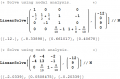Homework: Determind if the dependent source absorbs or supplied power to the circuit

michgkou

Joined Oct 3, 2019
41

Jony130

Joined Feb 17, 2009
5,292
You know what, none of your two solutions is correct. Why? Because you made the same error in your hand calculation and in LTspice. In simply words your "definition" of an I3 (and Vx voltage) current is wrong.

How can I3 be equal to I3 = (Vc - 9V) /1Ω?

Do you see the error?

Last edited:

michgkou

Joined Oct 3, 2019
41
You know what, none of your two solutions is correct. Why? Because you made the same error in your hand calculation and in LTspice. In simply words your "definition" of an I3 (and Vx voltage) current is wrong.

How can I3 be equal to I3 = (Vc - 9V) /1Ω?

Do you see the error?
So the I3=(Vc+9-Vb)/1 ?

Jony130

Joined Feb 17, 2009
5,292
What is the voltage at the right side of a 1 Ohm resistor and what is the voltage at the left side of a resistor?

Jony130

Joined Feb 17, 2009
5,292
this is incorrect I3=(Vc+9-Vb)/1 (?)
Yes, this is incorrect.
Do you know that the voltage between points C and B is (Vc - Vb)?

•michgkou

Jony130

Joined Feb 17, 2009
5,292
I draw again the circuit making source transformation and help me.
No, you cannot do that. In this case, this is incorrect. Because of the fact that the "control voltage" (Vx) is not equal to Vc - Vb

In your circuit the Vx voltage is (Vc - (9+Vb))

•michgkou

The Electrician

Joined Oct 9, 2007
2,866
What did you get for a final result?

michgkou

Joined Oct 3, 2019
41

Attachments

• 619.7 KB Views: 2

The Electrician

Joined Oct 9, 2007
2,866
You might be interested in seeing how it can be done in very compact form using linear algebra techniques.

The network is solved using both nodal and mesh analysis.

For the nodal analysis, I included the non-essential node between the 9 volt source and the 1 ohm resistor.

For the mesh analysis, the meshes and currents are I1, the lower left mesh; I2, the lower right mesh; I3, the upper mesh.

From these two solutions, the current in the dependent source is I3, and the voltage across the dependent source is Vd-Va.